/

### Probability distributions: location (i.e. shift) and scale variants

The location and scale of any probability distribution can be adjusted by using the (linear) transform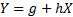where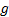and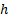are constants (adjusts the location, i.e. shifts the distribution, whilstadjusts its scale). This leaves the skew and (excess kurtosis) unaltered but alters the mean and variance as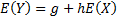and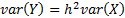.

In some cases the typical distributional specification already includes such components. For example, the normal distribution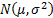is the location and scale adjusted version of the unit normal distribution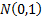.

In other cases the standard distributional specification does not include such adjustments. For example, the (standard) Student’s t distribution depends one just one parameter, its degrees of freedom. The probability distribution orientated Nematrian web functions recognise location and/or scale adjusted variants of wide range of standard probability distributions.